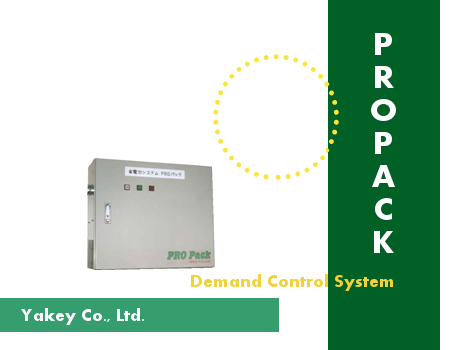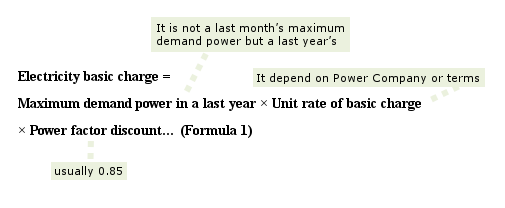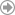TEL: 03-3727-5525 FAX: 03-3727-7565
Contact
• Home >
• Products >
• PRO Pack energy saving theory >
• PRO Pack energy saving theory①

# PRO Pack energy saving theory①PRO Pack energy saving theory① |  PRO Pack energy saving theory② |  PRO Pack energy saving theory③

#### Actual electricity energy contract

Large electric (contracted electricity over 50 kW) customers are supplied electricity based on Actual electricity energy contract. In this case, Power Company records the following data on a reading meter day.

①electric consumption per month (unit: kWh)
②maximum demand power on a month (unit: kWh)

Maximum demand power referred in ② is complicated and the explanation is as follows.

Firstly, a day is divided to 48 terms by 30 minutes (for example, 0:00～0:30, 0:30～1:00) and demand power is electric consumption per one term and there are 48 demand powers in a day. Furthermore, a period of time (a day, a month or a year) is set and the highest value of demand power in the time is called "maximum demand power". Maximum demand power on a month that shown by ② is measured from reading a meter day to a next month's. If electricity is actively used in 30 minutes on a month, this month's maximum demand power is high even total electric consumption is low. In Actual electricity energy contract, the electric charge is calculated by maximum demand power on a month.

#### The basic charge of Actual electricity energy contract

The method of calculation is the following formula.According to Formula 1, the electricity basic charge is increased proportion to the last year's demand power. The important thing is the maximum demand power is calculated by not a last month's but a last year's. In other words, the basic charge is fixed only 30 minutes in a last year.

#### Demand control

Demand control sets parameter (Called "demand parameter") to prevent using over a certain value. When electric consumption is liable to be actively used (over demand parameter value), an air conditioner that does not affect work (it is selected in advance) is automatically stopped and when demand power becomes less than demand parameter, it is again started. This is called "demand control".Return to the top of page